# Printable Number Grid 1-100Updated on Sep 16, 2022By Printablee Team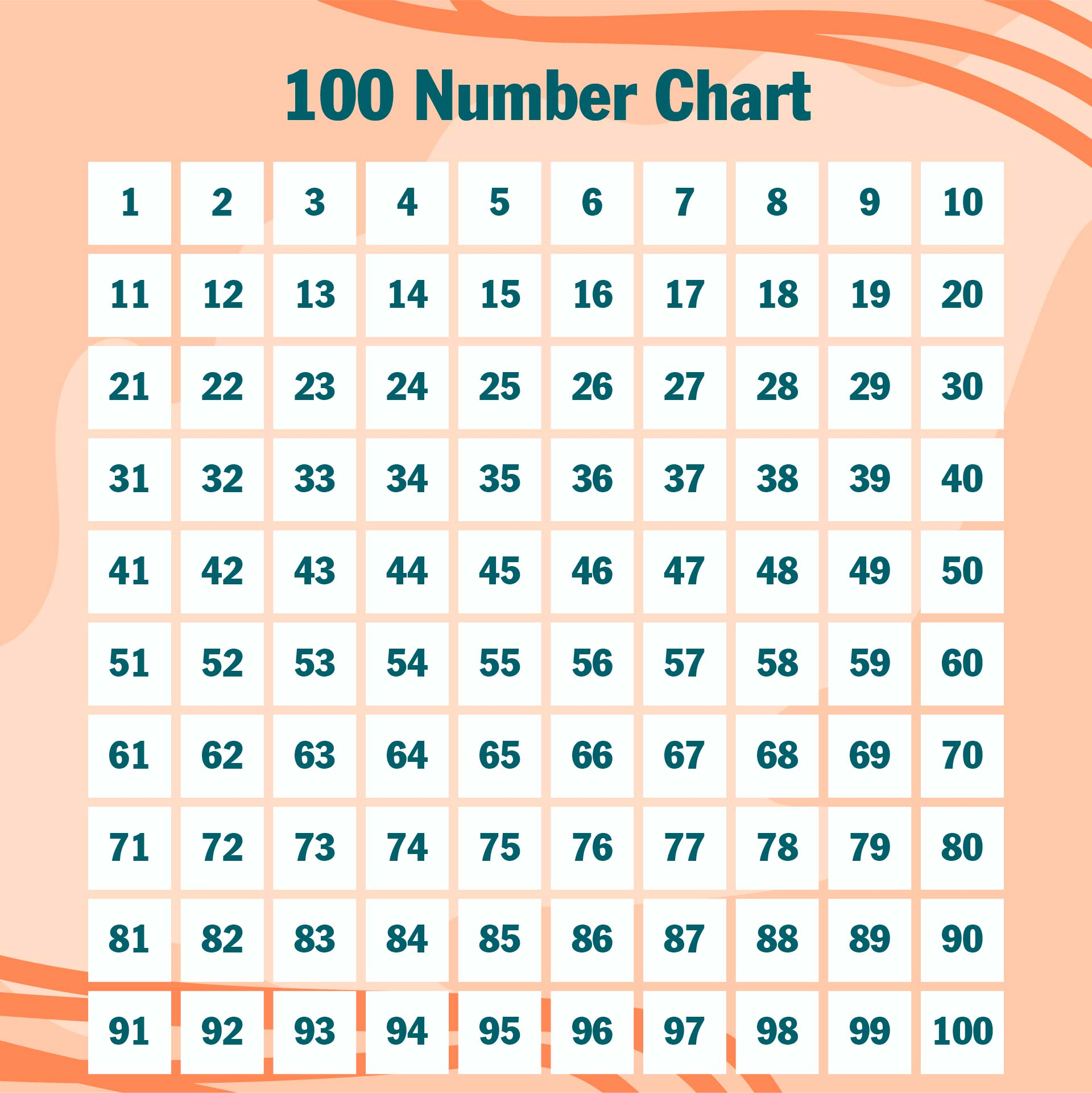### How do you construct a 1-100 number grid?

The numbers in a small column make up the number grid. In general, the number grid has ten columns in each row. The numbers are arranged in a way that can aid children's math learning. You can create a number grid by yourself or by printing a worksheet from the internet. The first step in completing the Do-It-Yourself number grid 1 to 100 is to prepare blank paper, markers, and rulers. Second, you can begin to create little columns across the top ten columns. Third, make a little column downward ten times. Fourth, once all of the columns have been completed, you may begin to write numbers. To the side are the numbers one, two, three, four, five, six, seven, eight, nine, and ten. Fifth, you write the following number, which is eleven under the number one, and so on until you reach the number one hundred. The column size can be adjusted to your preferences. If you wish to build 100 columns, use medium or small columns so that all of the numbers are on the same page and it is simpler to understand the grid later when studying. Another method for creating your own number grid is to print a page with the columns and then have the youngsters write the numbers from 1 to 100 on their own. The second way is simpler since it eliminates the need to line again to generate columns. When youngsters create their own number grid, they can learn how to write the correct numbers as well as how number patterns form. When writing numbers in the grid, parents should double-check the numbers or look up lessons on how to write numbers on the internet. If you need to use a number grid to learn addition and subtraction quickly, you can hunt for one that is already available on the web, download it, and print it right away. The worksheet number grid is then ready to use. In addition, at the bookstore, you may purchase a book containing a selection of number grid worksheets. This strategy can help make learning to utilize the number grid easier.

### How do I use the number grid 1 - 100?

The grid of numerals runs from one to one hundred. You can use this number grid to help you learn math. For example, if you want to locate the number 32, all you have to do is seek for a row of 30 and you'll see it. You must slide the tile to the right to utilize the number grid 1 – 100 for addition. If you want to compute a sum like 13 + 5, slide the box from 13 to the right five times in a row to get the result, which is 18. Let's try again, 46 plus 7, and the first thing you do is start at 46 and go to the right seven times in a row to get the number 53. The result is 46 plus 53. Now we'll look at another pattern, what if 4 plus 65. We cannot begin with 4 and then travel to the right 65 times before we may begin with the biggest number. Start from 65 and work your way to the right four times to find the number 69. As a result, 4 plus 65 = 69. Then we'll try a more challenging pattern, what if 56 plus 23. First, we begin with the largest addition, 56. Second, we note that number 23 contains two tens, thus we go down two columns from number 56. Third, we'll take a break at 76. Fourth, we note that the number 23 has three ones, thus we continue from the number 76 to the right three times to get the number 79. The sum of 56 and 23 is 79.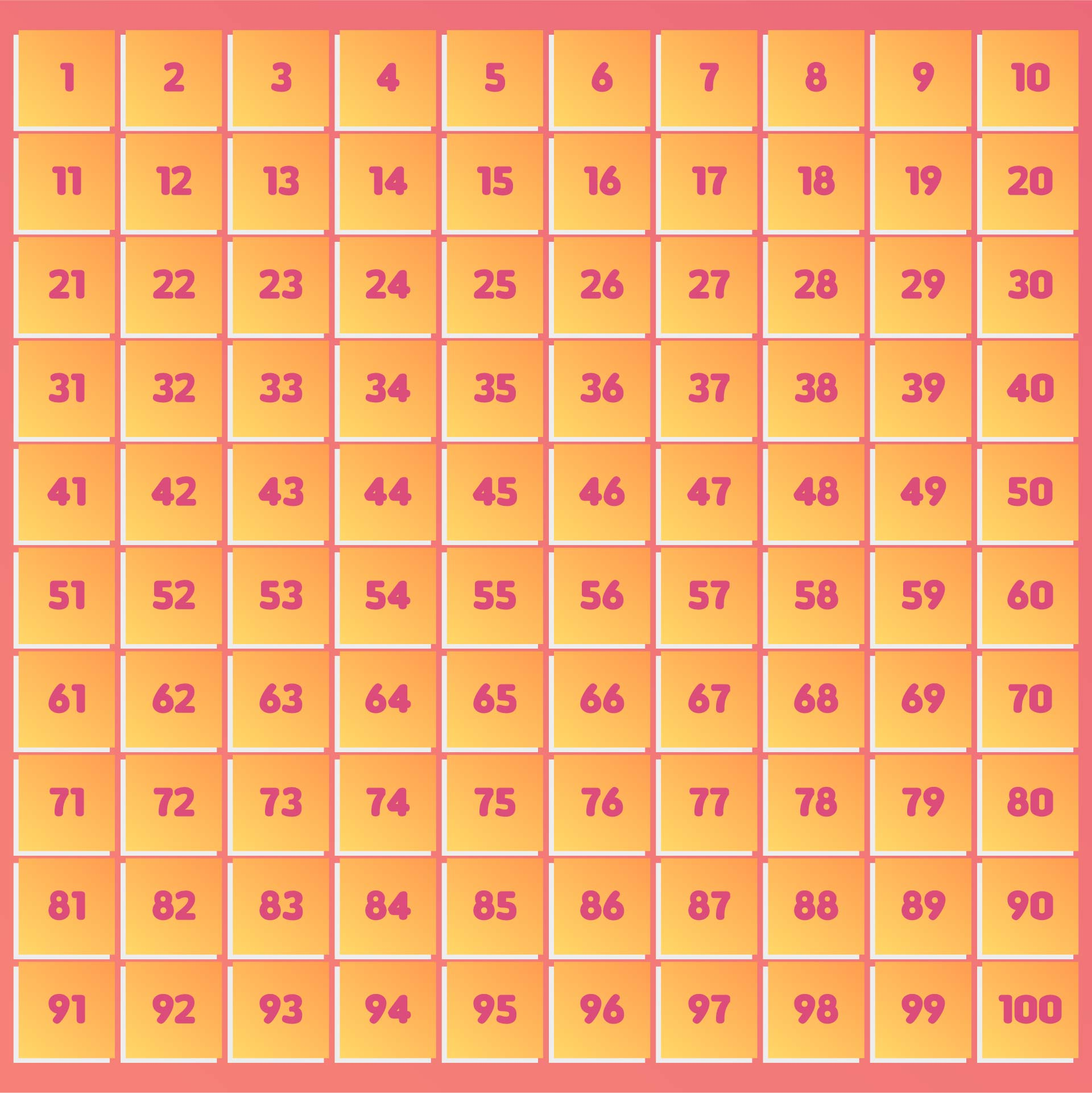We also have more printable number you may like:
Number Sheets 1 To 100 Printable
Number Tracing Worksheets Printable
Color By Number Printables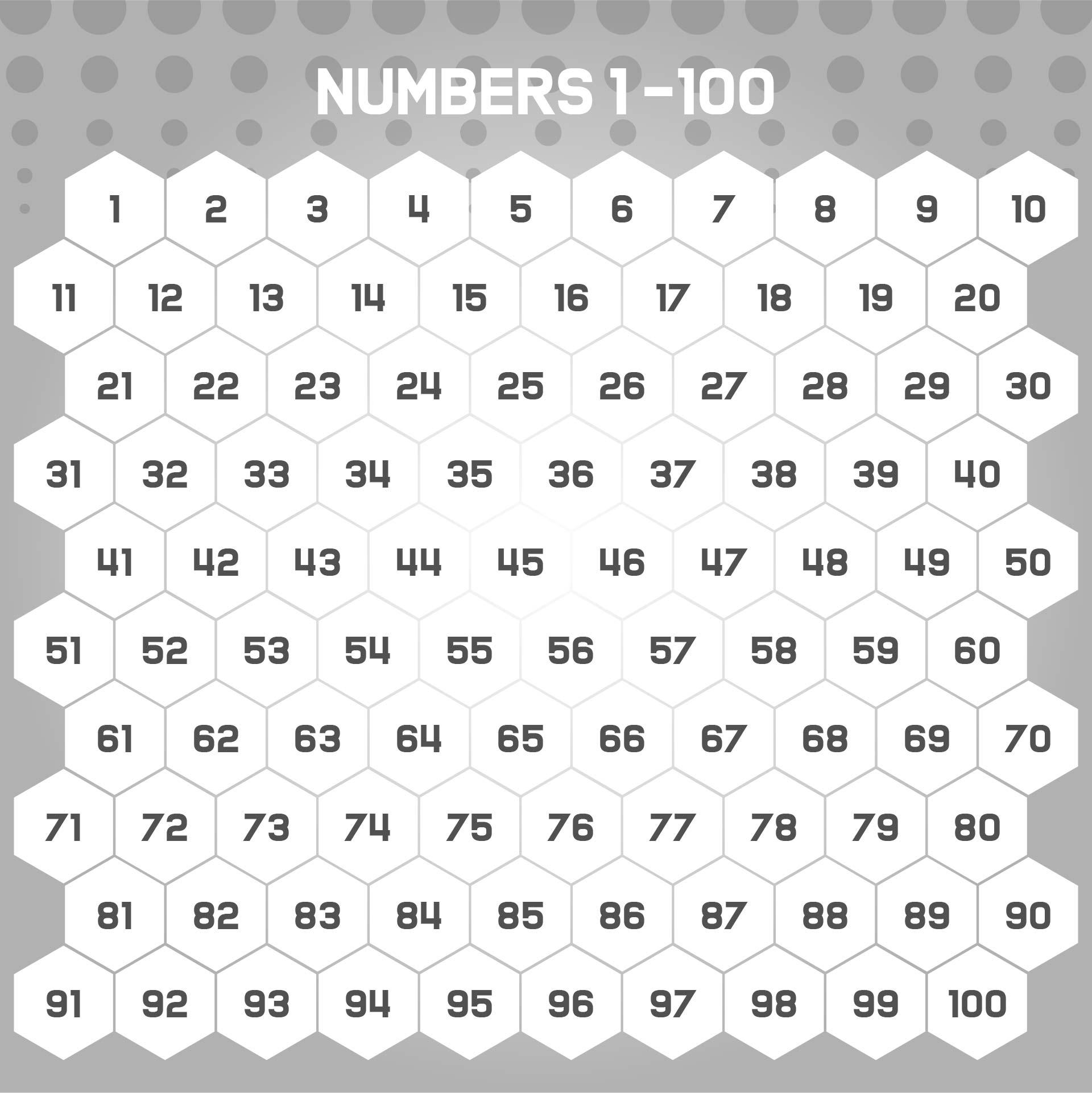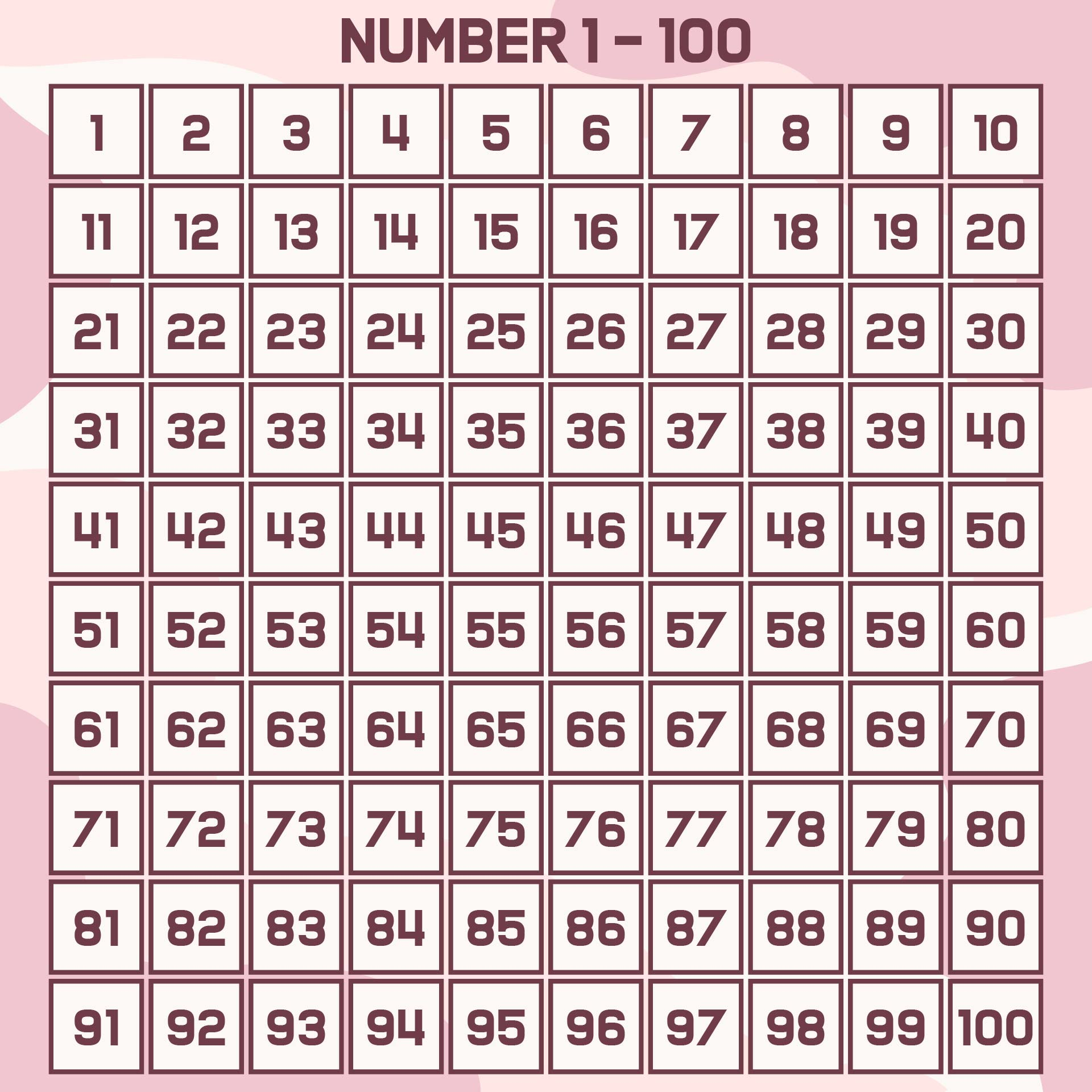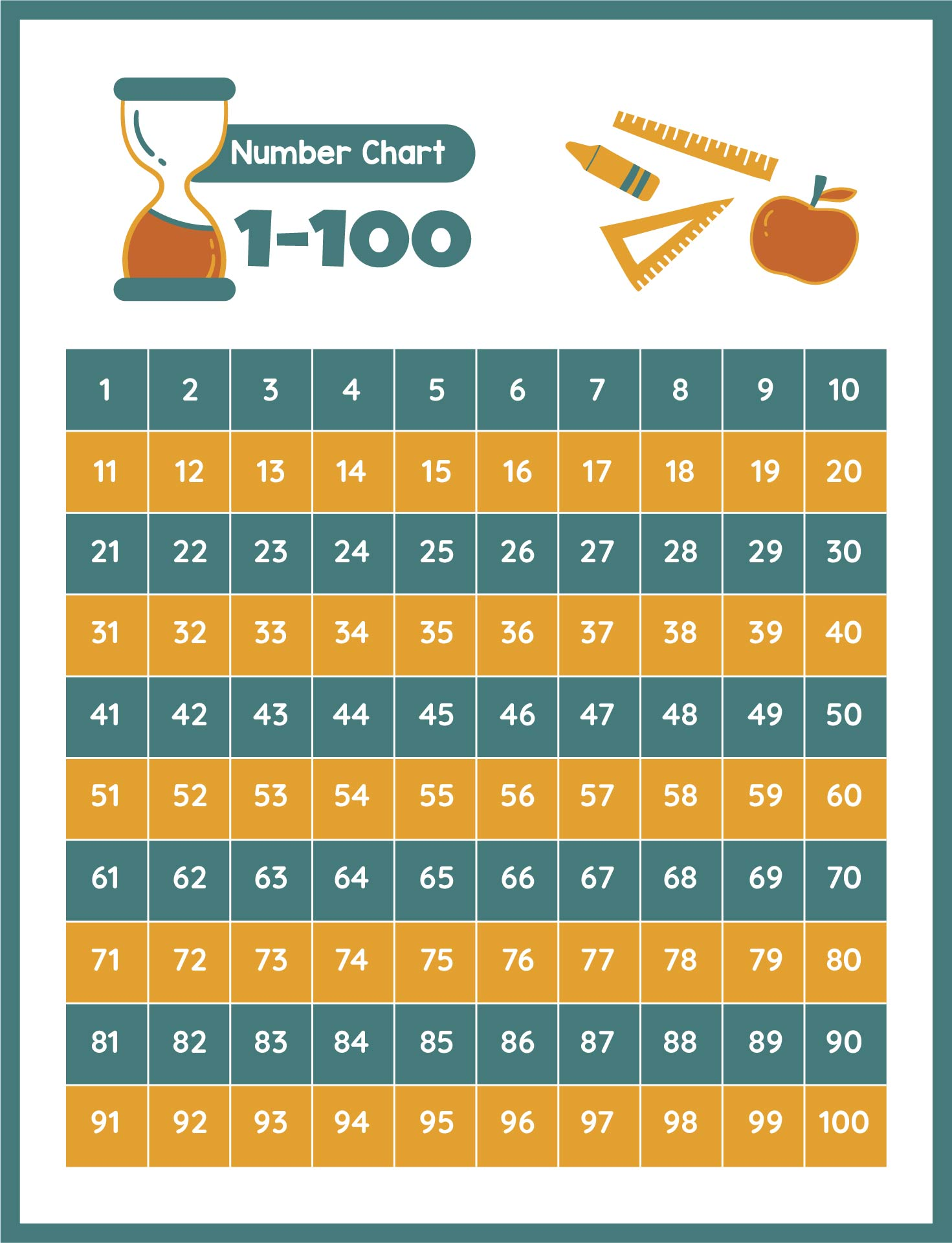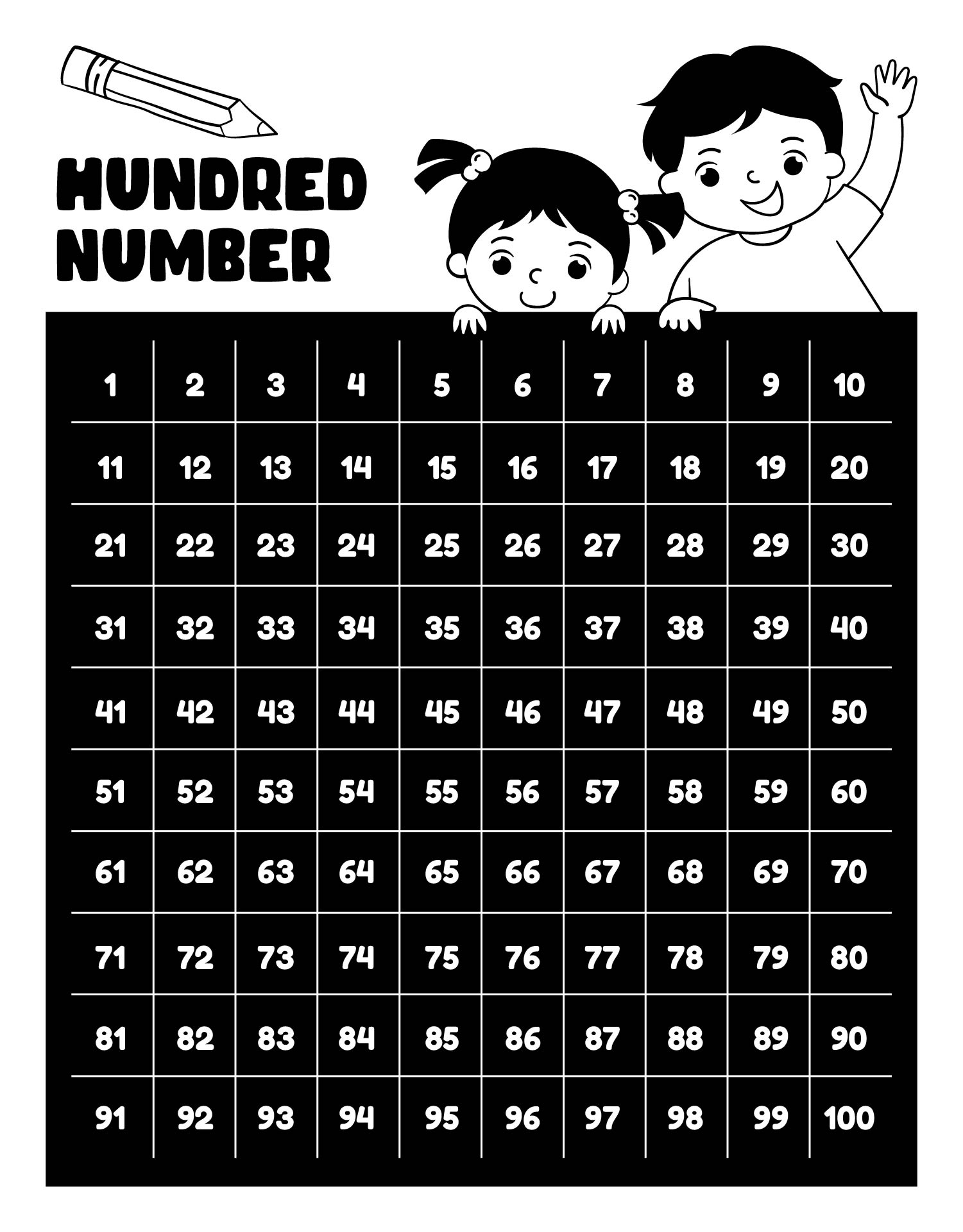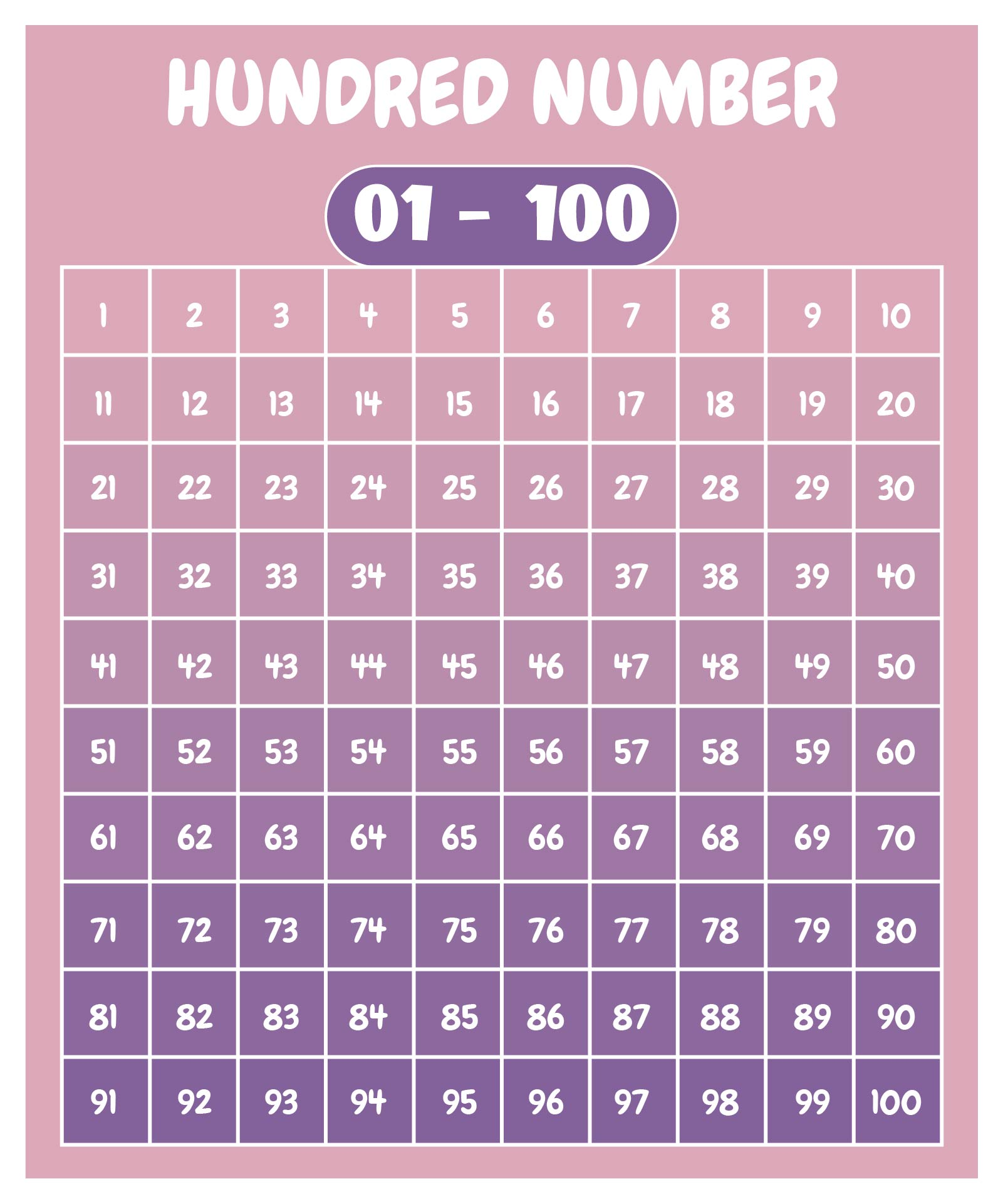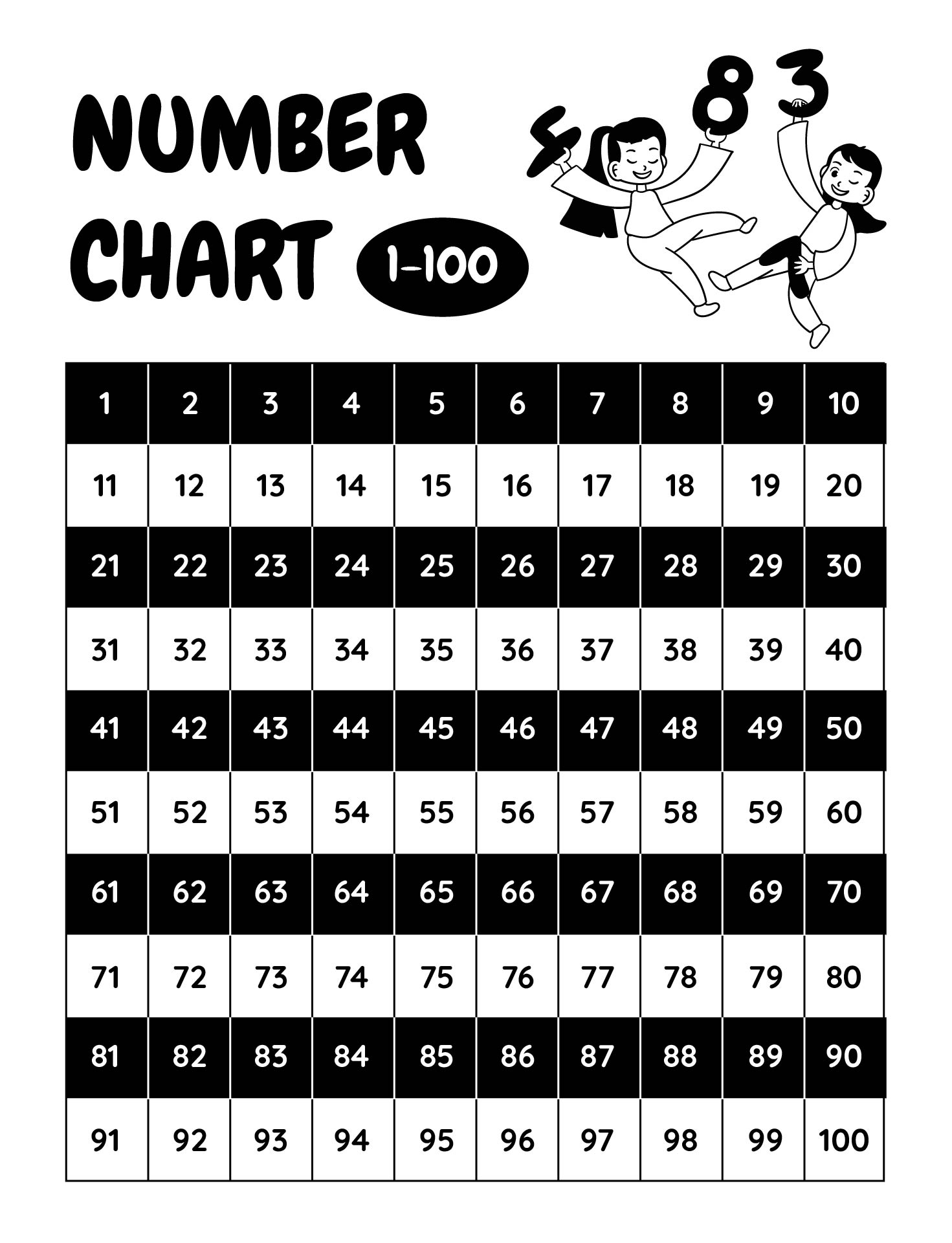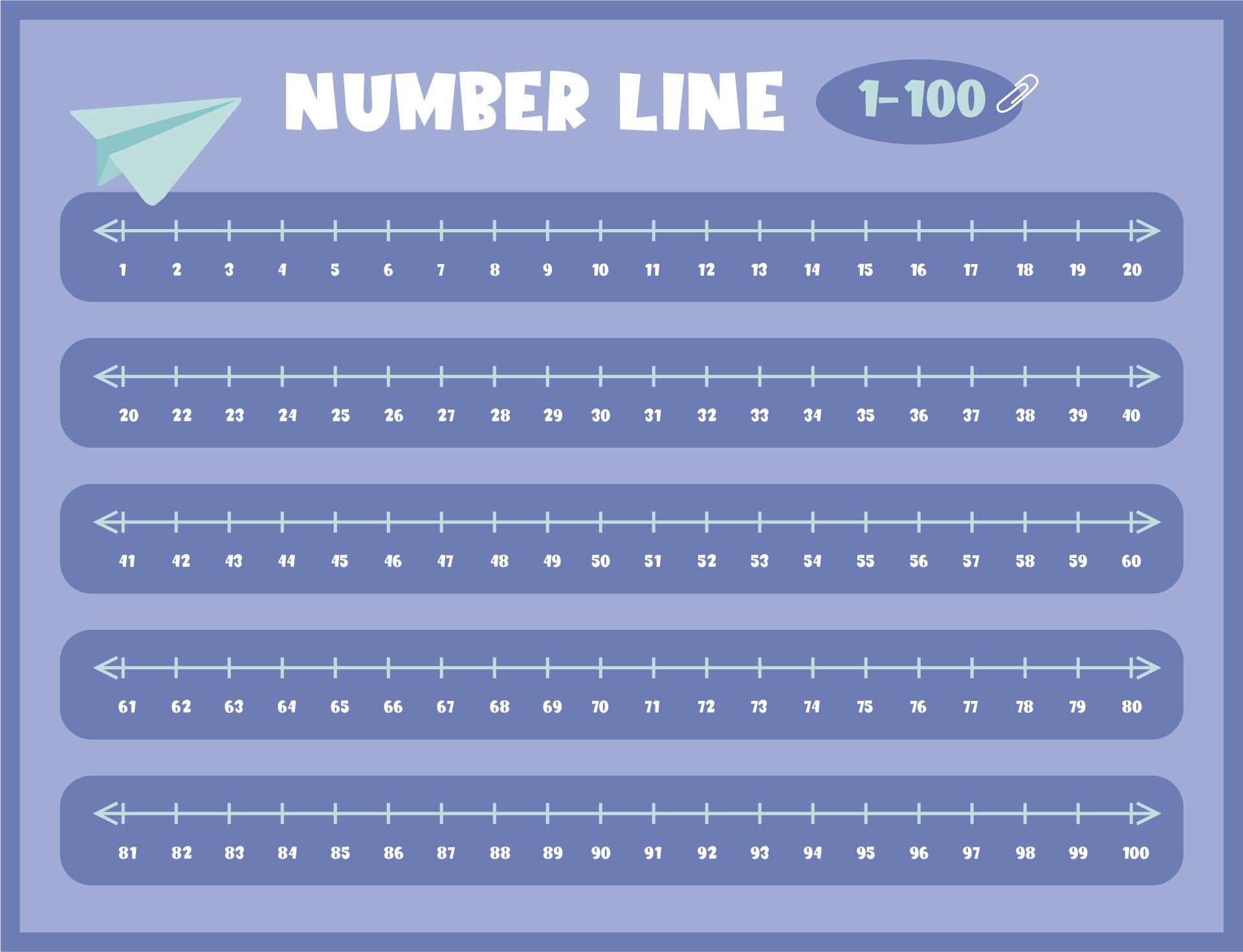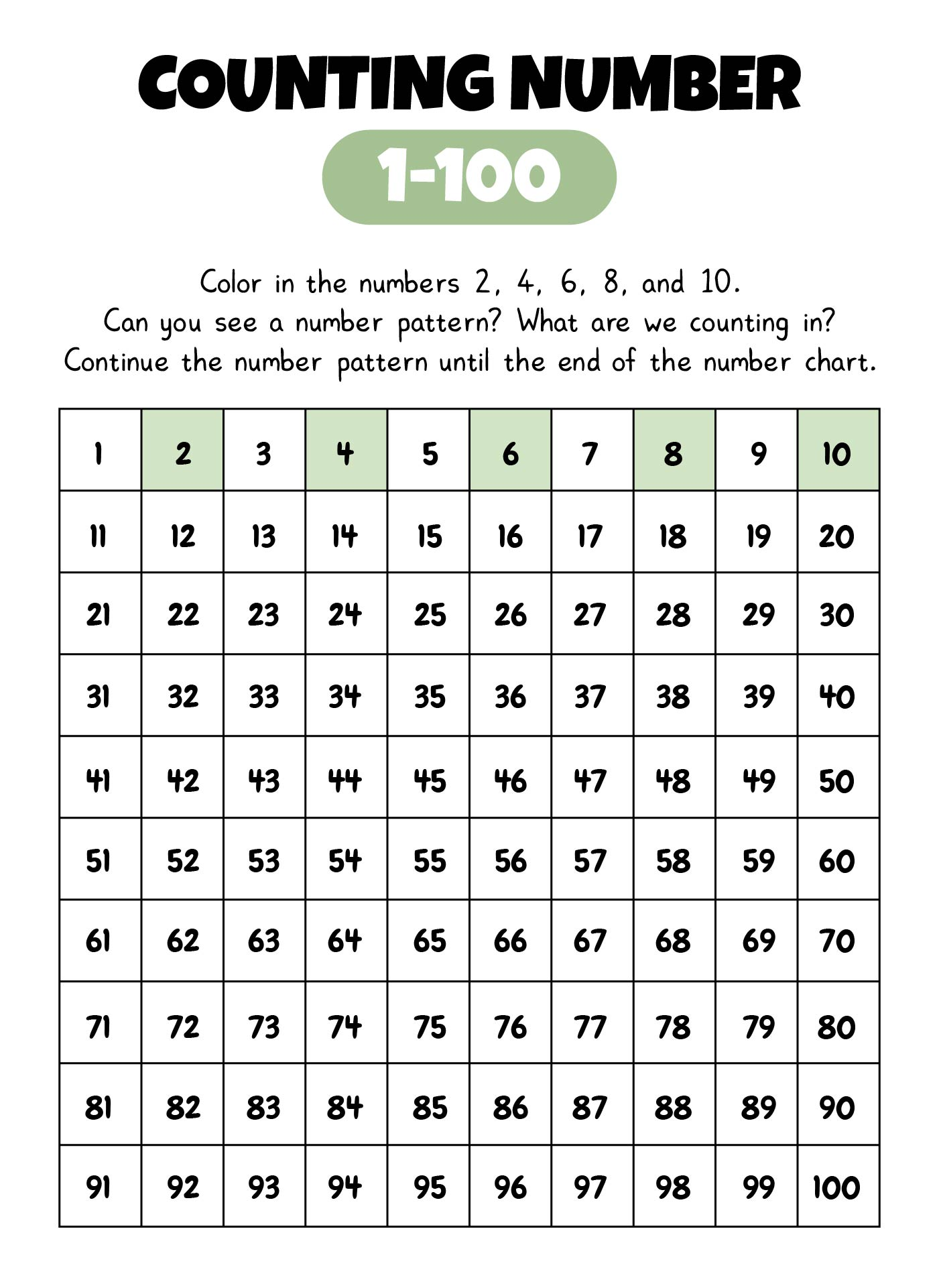### How do you utilize special tricks for the number grid 1 to 100?

You can use this grid to perform a few simple addition tricks. In addition, if I ask you to make adds like 36 plus 10, you don't need to manually calculate one by one like 37,38,39, 40,41,42,43,44,45,46, but you only need to scroll down to the column below it and you'll receive the result, which is 46. Let's try 30 plus 34 again. To answer this question, we can see that 30 is three tens, therefore we start at 36 and go down to the bottom column three times to get the number 66. So 30 plus 36 equals 66. Let's try something different: 25 plus 50. As we can see, 50 is 5 tens, so we start at 25 and work our way down to the bottom column five times. The result will be the number 75. As a result, 25 + 50 equals 75. That's simple, but keep in mind that this approach only works for numbers that are multiples of 10. If the number is less than or greater than ten, proceed to the right and down to the column below, as directed by the question.